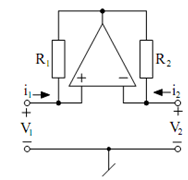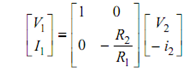## Negative Impedance Converter Assignment Help

Assignment Help: >> Basic Analog Circuits using Ideal Op-amps - Negative Impedance Converter

Negative Impedance Converter:

Negative-impedance converter (NIC) is an significant building block that is useful in realising negative impedances. A negative resistance is necessary an active device which may be used advantageously in realizing oscillators and enhancing the quality factor of resonators. The circuit realisation of the NIC is illustrated in Figure.Figure: Op-amp Realisation of the NIC

Routine analysis of the circuit gives

i1  = V1 - V0 /R1

i 2 = V2  - V0 /R2

Because of the property of the ideal op-amp

V1 = V2

From the above

i1/ i2  = R2 /R1

Therefore, the transmission matrix of the circuit is given byFrom the above transmission matrix, this is seen that the circuit is a NIC since upon terminating port 2 into a load impedance ZL, the impedance looking at the input and port is given by

Z in       =- (R1/R2  )Z L